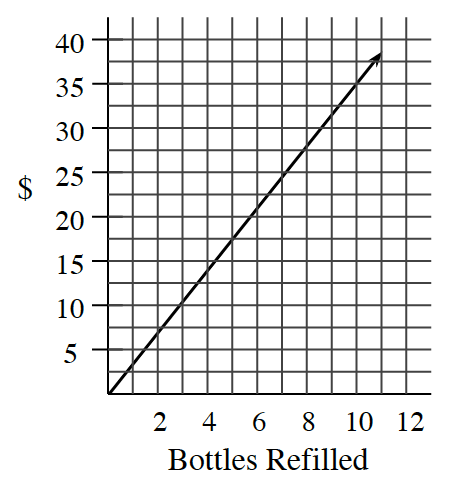### Home > ACC7 > Chapter 3 Unit 2 > Lesson CC3: 3.2.5 > Problem3-117

3-117.This problem is a checkpoint for unit rates and proportions. It will be referred to as Checkpoint 3.

In parts (a) through (c), use the given information to find the unit rate. In parts (d) through (f), write and solve a proportion based on the given information.

1. If $2\frac { 1 } { 4 }$ pounds of bananas cost $\1.89$, what is the cost per pound?

2. What is the weight per cm?

 weight (g) length (cm) $9$ $12$ $18$ $30$ $15$ $20$ $30$ $50$
3. Use the graph below to find the refill cost per bottle.4. If $200$ vitamins cost $\4.75$, what should $500$ vitamins cost?

5. If a basketball player made $72$ out of $85$ free-throw attempts, how many could she expect to make in $200$ attempts?

6. A cookie recipe uses $\frac { 1 } { 2 }$ teaspoon of vanilla with $\frac { 3 } { 4 }$ cup of flour. How much vanilla should be used with five cups of flour?

Check your answers by referring to the Checkpoint 3 materials located at the back of your book.

Ideally, at this point you are comfortable working with these types of problems and can solve them correctly. If you feel that you need more confidence when solving these types of problems, then review the Checkpoint 3 materials and try the practice problems provided. From this point on, you will be expected to do problems like these correctly and with confidence.

Answers and extra practice for the Checkpoint problems are located in the back of your printed textbook or in the Reference Tab of your eBook. If you have an eBook for Core Connections, Course 3, login and then click the following link: